# How to Supercharge Excel With Python

• 2477

How to Supercharge Excel With Python .I think you would agree that this is a nifty little library. If you are like me and you much prefer to work in Python rather than VBA but need to work in spreadsheets, then this can be an exceptional tool.

As much as Excel is a blessing, it is also a curse. When it comes to smallish enough data and simple enough operations Excel is king. Once you find yourself endeavouring outside of those zones however, it becomes a pain. Sure enough, you can use Excel VBA to get around such issues, but in the year 2020, you can thank your lucky stars because you don’t have to!

If only there was a way to integrate Excel with Python to give Excel… wings! Well now there is. A python library called xlwings allows you to call Python scripts through VBA and pass data between the two.

## Why integrate Python with Excel VBA?

The truth of the matter is, you can pretty much do anything in VBA. So, if that is the case, why would you want to use Python? Well, there are a number of reasons.

1. You can create a custom function in Excel without learning VBA (if you don’t know it already)
2. Your users are comfortable in Excel
3. You can speed up your data operations significantly by using Python
4. There are libraries for just about anything in Python (Machine Learning, Data Science, etc)
5. Because you can!!!

## Getting Set Up to Use xlwings

The first thing we need to do, as with any new library we want to use, is to install it. It’s super easy to do; with two commands we’ll be set up in no time. So, go ahead and type in your terminal:

``````pip install xlwings
``````

Once the library has been downloaded and installed, we need to install the Excel integration part. Ensure you’ve closed down all your Excel instances and in any terminal type:

``````xlwings addin install
``````

Assuming you experience no errors, you should be able to proceed. However, oftentimes on Win10 with Excel 2016, people will see the following error:

``````xlwings 0.17.0
[Errno 2] No such file or directory: 'C:\\Users\\costa\\AppData\\Roaming\\Microsoft\\Excel\\XLSTART\\xlwings.xlam'
``````

If you are one of the lucky ones to experience the above error, all you need to do is create the missing directory. You can do that easily by using the mkdir command. In my case, I did:

``````mkdir C:\\Users\\costa\\AppData\\Roaming\\Microsoft\\Excel\\XLSTART
``````

Assuming the successful installation of the excel integration with the python library, the main difference you will immediately notice is in Excel: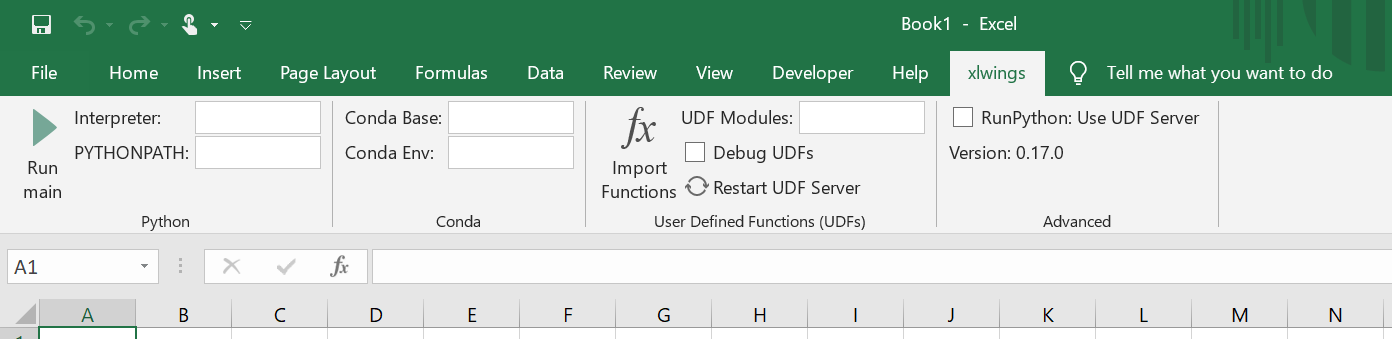## Enabling User Defined Functions for xlwings

First up, we need to load the Excel Add-in. You can hit Alt, L, H and then navigate to the directory above to load the plugin. Once you’re done, you should be able to see the following: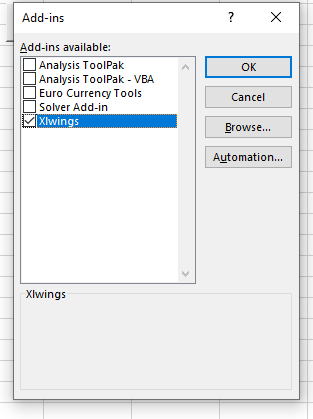Finally, you need to Enable Trust access to the VBA project object model. You can do that by navigating to File > Options > Trust Center > Trust Center Settings > Macro Settings: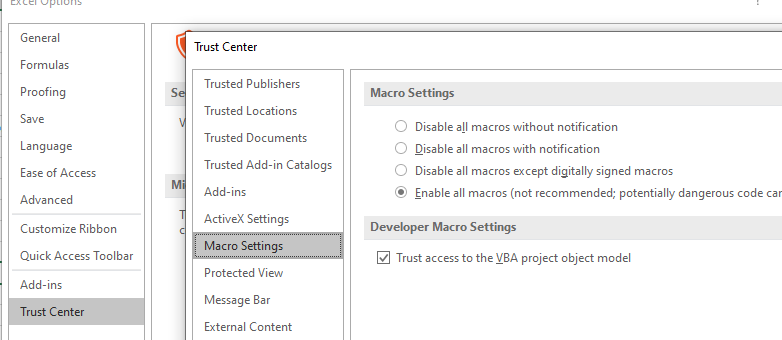## Getting Started with xlwings

There are two main ways you can go from Excel to Python (and back). The first one is to call a Python script directly from VBA, while the other one is through a User Defined Function. Let us have a quick look at both.

In order to avoid any confusion and to have the correct set up every time, xlwings offers to create your Excel spreadsheet, ready to go. Let us then use this functionality. Using the terminal, we navigate to the directory we like and type:

``````xlwings quickstart ProjectName
``````

I am calling this MyFirstPythonXL. The above command will create a new folder in your pre-navigated directory with an Excel worksheet and a python file.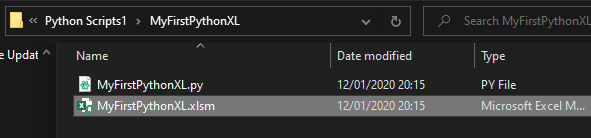Opening the .xlsm file, you immediately notice a new Excel sheet called _xlwings.conf. Should you wish to override the default settings of xlwings, all you have to do is rename this sheet and remove the starting underscore. And with that, we are all set up and ready to begin using xlwings.

## VBA to Python

Before we jump into the coding, let us first ensure we are all on the same page. To bring up our Excel VBA editor, hit Alt + F11. This should return the following screen: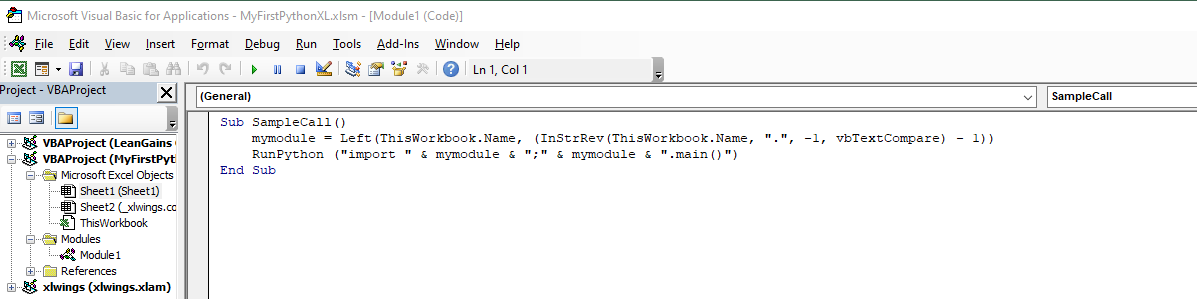The key things to note here is that this code will do the following:

1. Look for a Python Script in the same location as the spreadsheet
2. Look for a Python Script with the same name as the spreadsheet (but with a .py extension)
3. From the Python Script, call the function “main()”

Without any further ado, let us look at a few examples of how this can be used.

## Example 1: Operate Outside of Excel, and return the Output

In this example, we will see how you carry operations outside of Excel, but then return your results in the spreadsheet. This can have an infinite amount of use cases.

We will source data from a CSV file, do a modification on said data, and then pass the output to Excel. Let’s review how easy it is:

First up, the VBA code:

I have left this completely unchanged from the default.

Then, the Python code:

``````import xlwings as xw
import pandas as pd
def main():
wb = xw.Book.caller()
df['total_length'] =  df['sepal_length_(cm)'] + df['petal_length_(cm)']
wb.sheets.range('A1').value = df
``````

Which results in the following: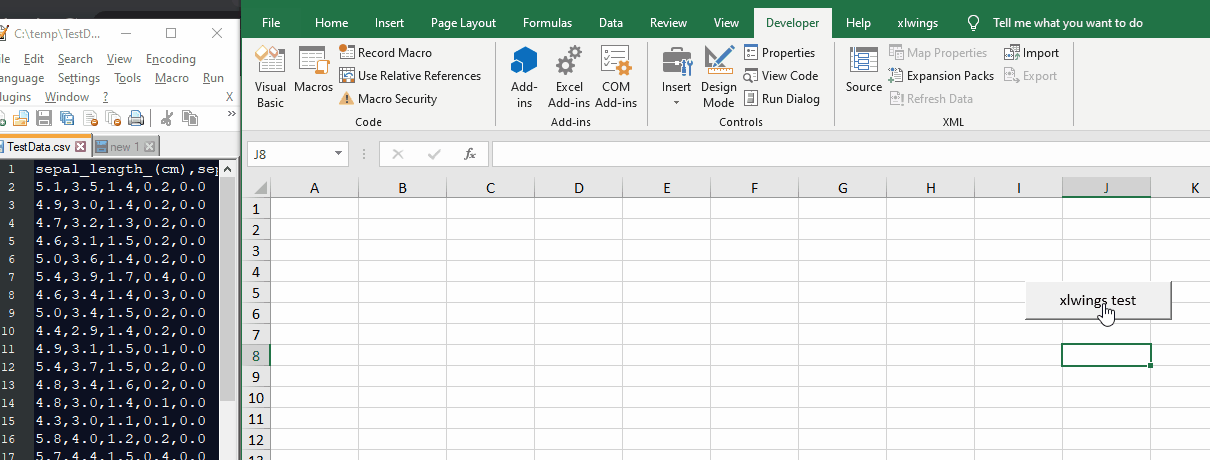xlwings in action

## Example 2: Use Excel Inputs to Drive the Operations

In this example, we will read inputs from Excel, do something with it in Python, and then pass the result back to Excel.

More specifically, we are going to read a Greeting, a Name and a file location of where we can find jokes. Our Python script will then take a random line from the file, and return us a joke.

First up, the VBA code:

I have left this completely unchanged from the default.

Then, the Python code:

``````import xlwings as xw
import random
def random_line(afile):
line = next(afile)
for num, aline in enumerate(afile, 2):
if random.randrange(num): continue
line = aline
return line
'Function from: stackoverflow
def main():
wb = xw.Book.caller()
listloc = str(wb.sheets.range('B3').value)
fhandle = open(listloc, encoding = 'utf-8')
wb.sheets.range('A5').value = wb.sheets.range('B2').value + ' ' + wb.sheets.range('B1').value + ' here is a joke for you'
wb.sheets.range('A6').value = random_line(fhandle)
``````

Which gives us: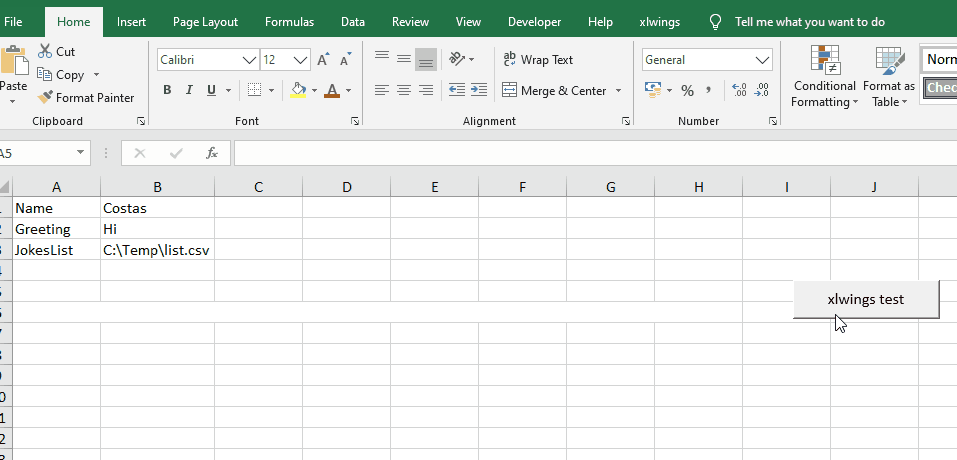## User-Defined Functions with xlwings

In pretty much the same fashion as before, we will be changing the code in the python file. In order to turn something into an Excel User Defined Function, all we need to do is include ‘@xw.func’ before the line the function is on:

The Python code:

``````import xlwings as xw
@xw.func
def joke(x):
wb = xw.Book.caller()
fhandle = open(r'C:\Temp\list.csv')
for i, line in enumerate(fhandle):
if i == x:
return(line)
``````

The result: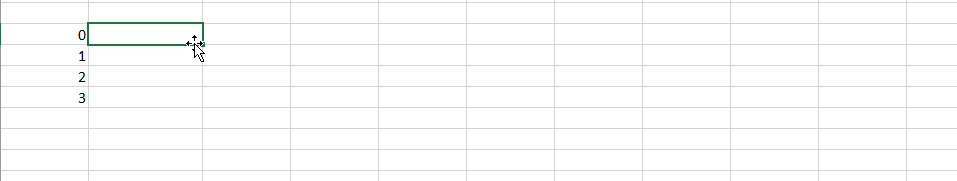## Conclusion

I think you would agree that this is a nifty little library. If you are like me and you much prefer to work in Python rather than VBA but need to work in spreadsheets, then this can be an exceptional tool.